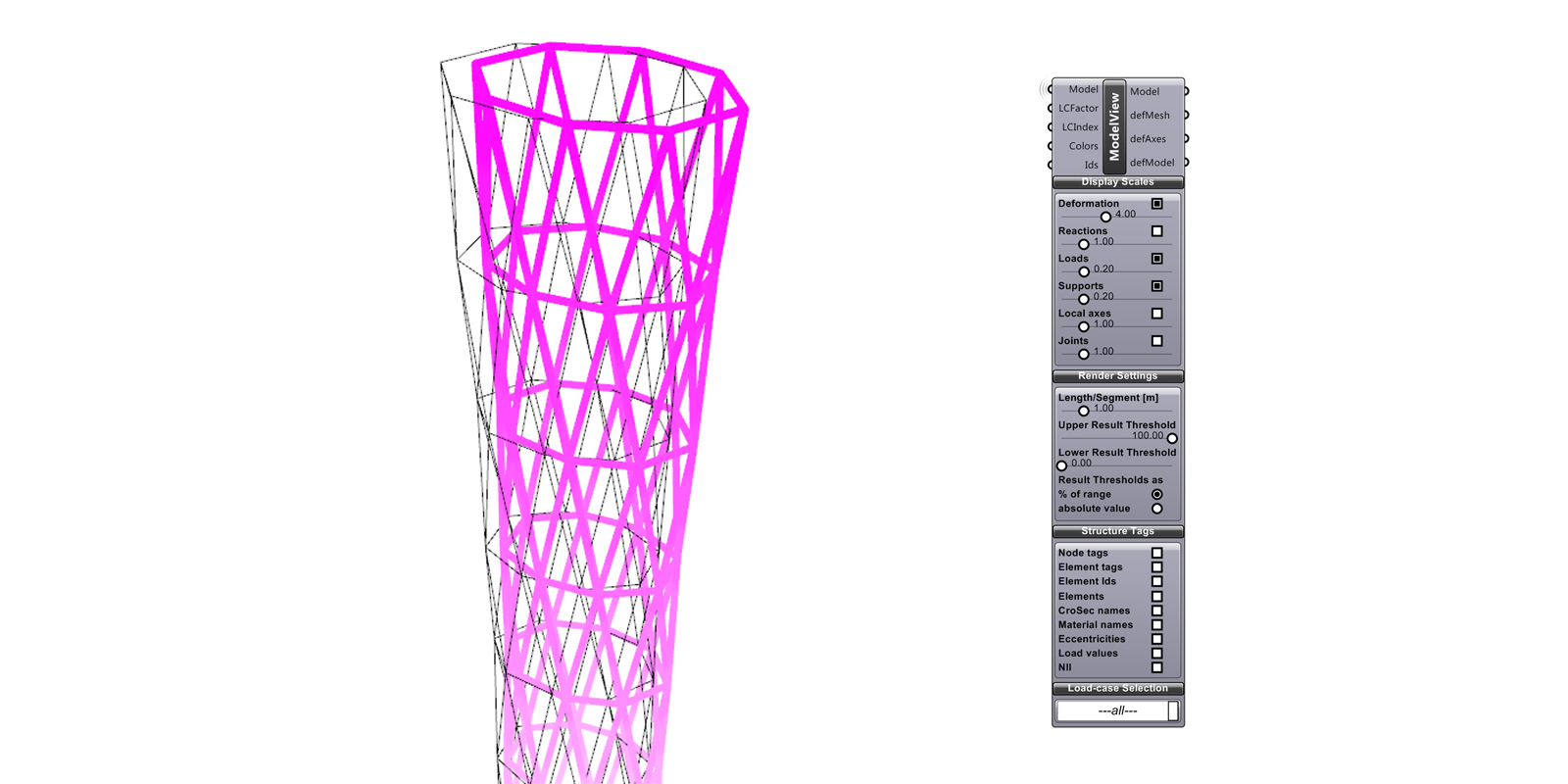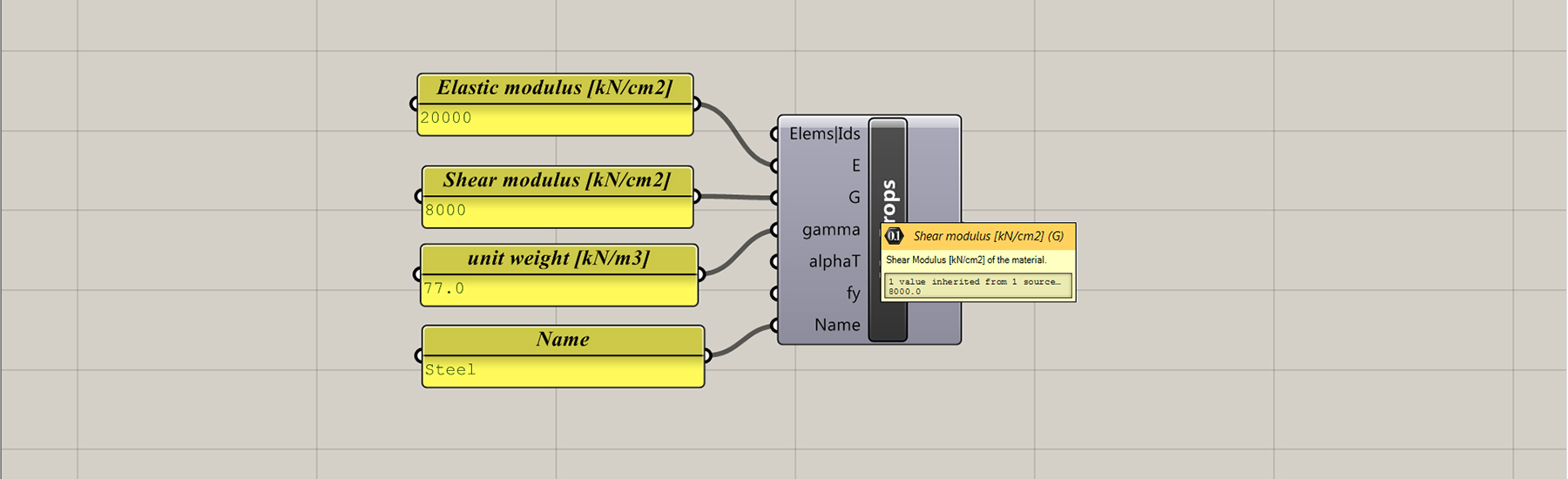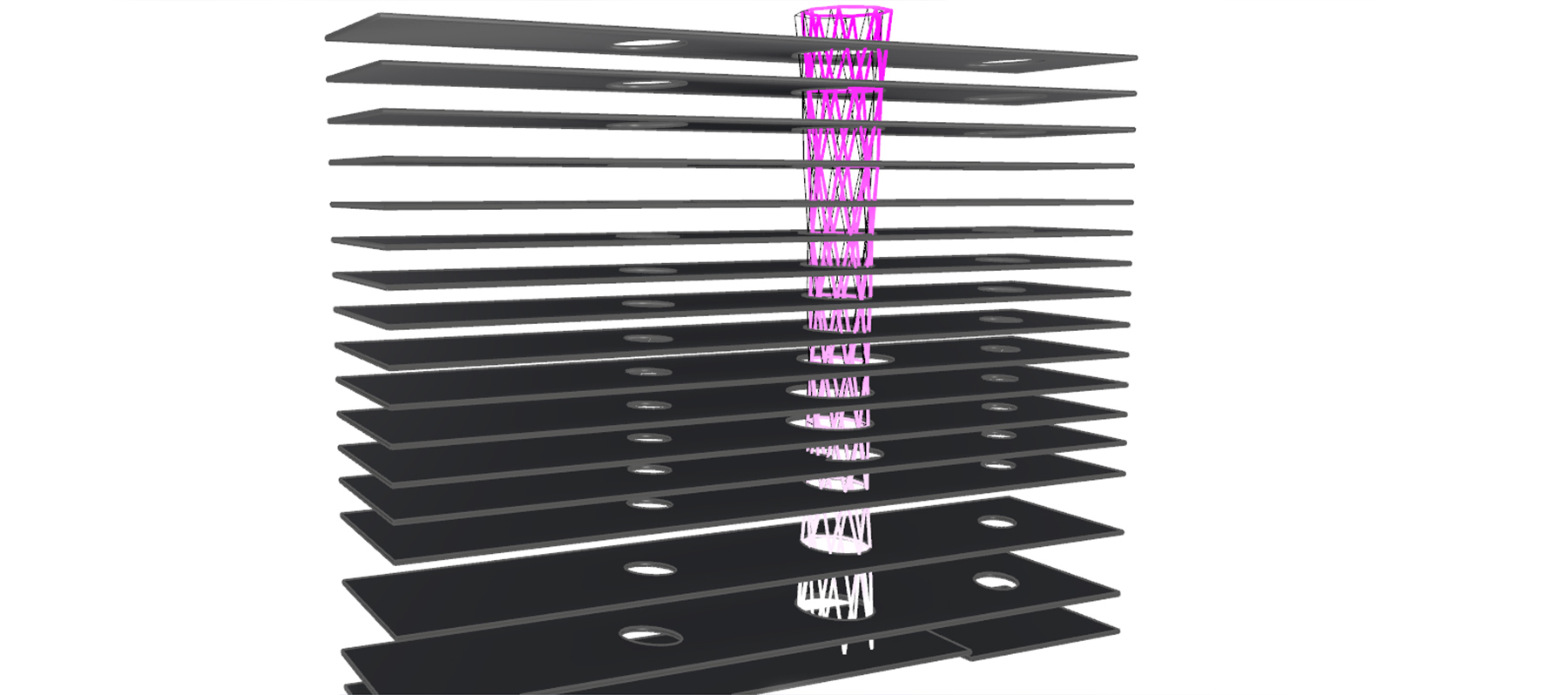# Structural analysis

Karamba is an interactive, parametric finite element program that lets you analyse the response of 3-dimensional beam and shell structures under arbitrary loads within Grasshopper. Karamba is being developed by Clemens Preisinger in cooperation with Bollinger-Grohmann-Schneider ZTGmbH Vienna.Karamba has a fixed set of units either SI or Imperial. For SI units it expects the model geometry to be in metres (m), the structural member dimensions in centimetres (cm) and loads in kilonewton (kN). Displacement are returned in meters (m). This mixing of units can be confusing. The kind of physical units that Karamba components expect to receive show up in the tool-tip which appears when the mouse pointer hoovers over an input-plug.There are 6 basic steps in using Karamba:

1. Create wire-frame geometry
2. Convert wire-frame geometry to Karamba beams
4. Assemble the structural model with points, elements, supports and loads
5. Analyse the structural model
6. View the analysed model

In this example we were interested in optimising the size and rotation of the ‘structural tubes’ based on minimising the lateral displacement. (For more information on the geometric setup of the diagrid structure, refer to this tutorial). The workflow is as follows:• The centre line geometry of the diagrid is first fed into the ‘LineToBeam’ component.
• The bottom points of the diagrid are extracted and used as the supports.
• The loads are then calculated. Since this script is only interested in lateral displacement and not say gravity, a wind load of 2750kN is applied evenly over the analytical points/nodes. This force is a vector in the Y axis.
• The cross sections and material properties of the structural tubes are defined. In this instance we are using a 35.5 CHS with 1.2cm thickness. Attention is required to ensure the correct units are entered into the ‘MatProps’ component.
• The structural model is then assembled and visualised.
• The maximum lateral displacement is then extracted and analysed to determine the fitness of the solution.Note that with the ‘ModelView’ component, the display scales can be modified. In the example below the deformation scale is set to 4.0 to exaggerate the displacement for clarity.